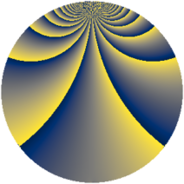# Properties

 Label 361.2.iLevel $361$ Weight $2$ Character orbit 361.i Rep. character $\chi_{361}(7,\cdot)$ Character field $\Q(\zeta_{57})$ Dimension $1080$ Newform subspaces $1$ Sturm bound $63$ Trace bound $0$

# Related objects

## Defining parameters

 Level: $$N$$ $$=$$ $$361 = 19^{2}$$ Weight: $$k$$ $$=$$ $$2$$ Character orbit: $$[\chi]$$ $$=$$ 361.i (of order $$57$$ and degree $$36$$) Character conductor: $$\operatorname{cond}(\chi)$$ $$=$$ $$361$$ Character field: $$\Q(\zeta_{57})$$ Newform subspaces: $$1$$ Sturm bound: $$63$$ Trace bound: $$0$$

## Dimensions

The following table gives the dimensions of various subspaces of $$M_{2}(361, [\chi])$$.

Total New Old
Modular forms 1152 1152 0
Cusp forms 1080 1080 0
Eisenstein series 72 72 0

## Trace form

 $$1080 q - 38 q^{2} - 19 q^{3} - 8 q^{4} - 37 q^{5} - 38 q^{6} - 40 q^{7} - 38 q^{8} + 49 q^{9} + O(q^{10})$$ $$1080 q - 38 q^{2} - 19 q^{3} - 8 q^{4} - 37 q^{5} - 38 q^{6} - 40 q^{7} - 38 q^{8} + 49 q^{9} + 19 q^{10} - 40 q^{11} - 38 q^{12} - 38 q^{13} - 38 q^{15} - 8 q^{16} - 94 q^{17} - 38 q^{18} - 38 q^{19} - 46 q^{20} - 38 q^{21} - 19 q^{22} - 34 q^{23} - 30 q^{24} - 7 q^{25} + 41 q^{26} - 76 q^{27} - 15 q^{28} + 60 q^{30} - 38 q^{31} - 38 q^{32} - 114 q^{33} - 38 q^{34} - 33 q^{35} - 38 q^{37} - 76 q^{38} + 26 q^{39} - 38 q^{40} - 38 q^{41} - 32 q^{42} + 43 q^{43} - 26 q^{44} + 47 q^{45} + 26 q^{47} - 456 q^{48} - 32 q^{49} + 38 q^{50} + 38 q^{51} - 38 q^{52} - 38 q^{53} - 214 q^{54} - 29 q^{55} + 304 q^{56} - 38 q^{57} - 46 q^{58} - 38 q^{59} - 33 q^{61} - 13 q^{62} + 68 q^{63} - 110 q^{64} + 38 q^{65} - 26 q^{66} - 38 q^{67} - 96 q^{68} - 228 q^{69} - 342 q^{70} - 38 q^{71} - 38 q^{72} - 29 q^{73} + 50 q^{74} + 190 q^{75} + 323 q^{76} - 60 q^{77} + 228 q^{78} - 38 q^{79} + 322 q^{80} + 129 q^{81} + 48 q^{82} - 35 q^{83} + 19 q^{84} - 23 q^{85} - 684 q^{86} + 37 q^{87} - 38 q^{88} + 76 q^{89} - 722 q^{90} - 114 q^{91} - 22 q^{92} - 30 q^{93} + 228 q^{94} - 152 q^{95} + 142 q^{96} + 19 q^{97} + 57 q^{98} + 2 q^{99} + O(q^{100})$$

## Decomposition of $$S_{2}^{\mathrm{new}}(361, [\chi])$$ into newform subspaces

Label Dim $A$ Field CM Traces $q$-expansion
$a_{2}$ $a_{3}$ $a_{5}$ $a_{7}$
361.2.i.a $1080$ $2.883$ None $$-38$$ $$-19$$ $$-37$$ $$-40$$